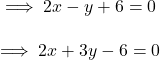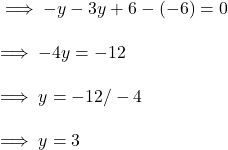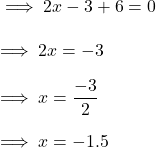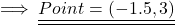## Find the intersection point between the lines of equations: 2x-y+6=0 and 2x+3y-6=0 ​

Question

Find the intersection point between the lines of equations:

2x-y+6=0 and 2x+3y-6=0 ​

in progress 0
6 months 2021-08-02T07:27:58+00:00 2 Answers 2 views 0

1. Step-by-step explanation:

The two equation will intersect each other at the point which will be the solution of the given two equations , and the given equations are ,On subtracting the given equations we have,Put this value in any equation , we have ,Hence the lines will Intersect at ,2. for the first one x = 1/2 y-3″ and y
=
2
x
+
6 and for the other one is x
=  −
3
/2  y+
3  and y=

2
/3  x
+
2

how i did this Step 1: Add -3y to both sides.

Step 2: Add 6 to both sides.

Step 3: Divide both sides by 2.